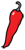The Grand Locus / Life for statistical sciences

## The Brownian labyrinthArchitecture and art show that human culture often uses the same basic shapes. Among them, labyrinth is an outsider for its complexity. Made famous by the Greek myth of Theseus and the Minotaur, labyrinths are found in virtually every culture and every era. The Wikipedia entry of labyrinth shows different designs, but they all have in common the intricate folding of a path onto itself, in such a way that the distance you have to walk inside the labyrinth is much larger than your actual displacement in space.

Fictions of all genres are also fraught with labyrinths. Perhaps one of the most vivid appearance of the labyrinth theme in literature is The Garden of Forking Paths by Borges. In this short story, Borges evokes a perfect labyrinth. Like in gamebooks, this special book that follows every possible ramification of the plot, and not just one. In some passages the hero dies, in some others he lives, in such a way that one can read the novel in infinitely many ways.

An invisible labyrinth of time. To me, a barbarous Englishman, has been entrusted the revelation of this diaphanous mystery. After more than a hundred years, the details are irretrievable; but it is not hard to conjecture what happened. Ts’ui Pe must have said once: I am withdrawing to write a book. And another time: I am withdrawing to construct a labyrinth. Every one imagined two works; to no one did it occur that the book and the maze were one and the same thing.

This passage illustrates the author's idea of the perfect labyrinth: an "invisible labyrinth of time". Bifurcations occur in time, not in space. Individuals are prisonners of their own invisible labyrinth. Every time they take a decision, they can explore only one path of the labyrinth of their life. With more freedom come more bifurcations, with more bifurcations comes less freedom to explore them. There is no esape from this pradox, neither from this labyrinth.

This paradox of constraint by freedom and the whole theme of The Garden of Forking Paths are reminiscent of a kind of mathematical monstruosity that is called random fractal. One of them in particular, the Brownian motion, has been fascinating mathematicians for almost a century. Initially described by a biologist observing the movement of pollen grains in water, it was much later constructed by pioneer mathematicians Wiener, Bachelier and Lévy.

It was recently brought to my attention that Einstein introduced the current interpretation of the Brownian motion as the diffusion of a microscopic particle in a fluid. Like the perfect maze of The Garden of Forking Paths, the countless collisions with other particles produce a random trajectory that keeps changing at every moment.

Such a description is hard to capture by pure mathematics, and even though it has been done, it relies on advanced theorems. However, Lévy proposed an equivalent construction of the Brownian motion that gives intuitive understanding of some of its properties.

You start by drawing a segment. You then take the middle of that segment and move it a little bit at random, so that you get a broken line with two segments. You take the middles of these two segments and move them again at random, so that you obtain a broken line with 4 segments. If you repeat the process an infinite number of times, you obtain a random curve consisting of so many segments that it will be infinitely convoluted. This process produces a continuous curve, appearing to change infinitely, which is an intuitive description of fractals.

More technically, the principle of Lévy's construction, is to draw a line between the origin (0,0) and the point (1,x) where x is taken from a standard Gaussian distribution. In the subsequent steps of the construction, the midpoints are moved up or down by sampling a number from a Gaussian distribution with smaller and smaller variance. A random example of the first steps of Lévy's construction of the Brownian motion is shown below.The figure represents the first 9 steps of Lévy's construction. The process never stops in reality, but the screen resolution does not show any difference beyond step 9. You can see that the line becomes more and more jittered as more midpoints and random noise are added to the curve. In the following technical section I give the R code that I used to generate the figure.

Here is an R function that recursively constructs the trajectory of a Brownian motion. You can just copy it in your R session.

levy_next_step <- function(step_n=0) {	   # Initialize if needed.   if (length(step_n) == 1) {   # This is step_0.       return (c(step_n, rnorm(1, step_n, 1)));   }      # Else make sure 'step_n' has length 2^n + 1.   n <- log2(length(step_n)-1);   stopifnot(n == floor(n));      # Get the midpoints and add noise.   midpoints <- filter(step_n, rep(.5,2)) +       rnorm(2^n+1, sd=1/2^((n+2)/2));      # Convenience 'zip' function.   zip <- function(x, y) {      as.vector(rbind(x,y));   }      # Zip and return (but remove last point).   last_index <- 2^(n+1)+2;   return(zip(step_n, midpoints)[-last_index]);}

Now you can make your own Levy construction of the Brownian motion by typing

x <- 0x <- levy_next_step(x); plot(x, type='l', ylim=c(-1,1))x <- levy_next_step(x); plot(x, type='l', ylim=c(-1,1))x <- levy_next_step(x); plot(x, type='l', ylim=c(-1,1))...

Of course, you can just type 'up arrow' followed by 'enter' to see the construction of the random fractal.

There is a lot more to say about the Brownian motion and we may cross its path again in our future meanderings. For now, this is all I need to introduce the idea that p-values are crap... Stay tuned.